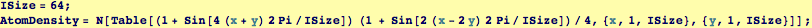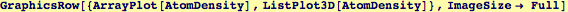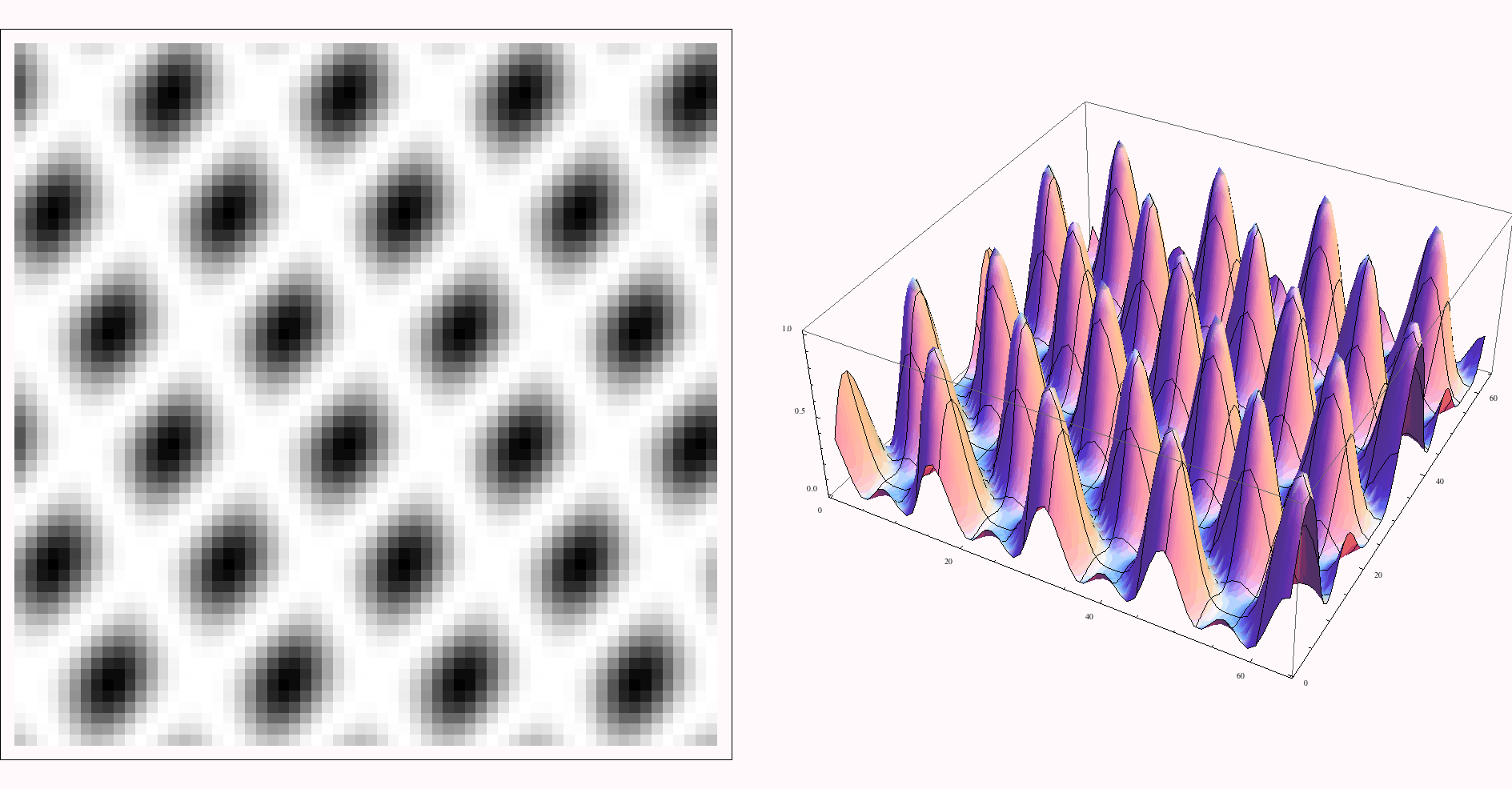Creating Sample Atomic Data and Lattices for Fourier Analysis and Diffaction Simulation.

Here we create an image such as might be seen in an transmission electron microscope. For our first set of  data, we use intererence of two sine waves to produce a simulation of the density of scattering centers in an atomic lattice.  Most of the physical aspects of atomic imaging and diffraction can be simulated with the two-dimensional techniques that are produced in these notes.We show the simplest visulations of our data, we will improve on the coloring methods below.  Here we show our two dimensional crystal as a gray-intensity map and with a three dimensional plot of scattering intensity over an x-y plane.Created by Wolfram Mathematica 6.0  (01 November 2007)